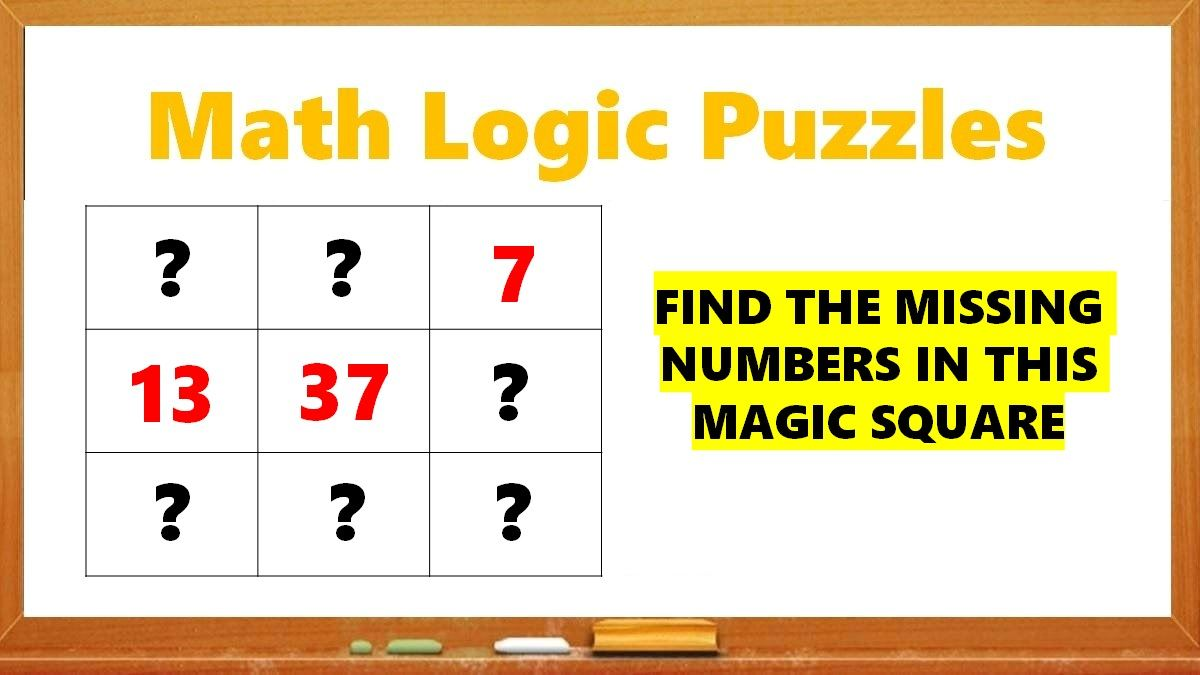# Math Riddles: Solve This Magic Square Puzzle in 20 Seconds

Math Riddles: This is a Magic Square. Only 1% of people with a high IQ can find the missing numbers in this tricky logic magic square puzzles in 20 seconds.Math Riddles: Solve This Magic Square Puzzle in 20 Seconds

Math Riddles are so challenging, but that makes them worthwhile to solve. Math riddles are logical problems that require strong analytical abilities, high IQ, knowledge of math concepts, and good calculation skills. Math riddles have also been known to make Mathematics enjoyable for kids, students, and even adults to solve. Riddles, puzzles, and brain teasers can develop strategic thinking, logical reasoning, and problem-solving skills.

Today, we bring you another math puzzle that is all over social media. These Tricky Logic Math Square Puzzle has attracted millions of answers but very few got it right.

Can you solve this hard math magic square puzzle? You have 20 seconds!

## Tricky Magic Square Solution

First, we understand that a 3 x 3 Magic Square is a square that has three rows and three columns. The sum of every row, column, and diagonal will equal to same magic number. In this magic square, the sum of all the rows, column, and diagonal should equal to 111.

Now, let us solve this magic square. We have three numbers to begin with. Find the step-by-step magic square solution below:

Now, we have the middle row figured out. We will now figure out the last column from the right with the information.

Now, we have figured out one row and one column. Using this information, we will solve a diagonal. See below:

We now shall solve another diagonal. See below:

Now, we have information in the first column from the left. We shall now solve the first row with the available information.

Wow, we have figured the first row too. All we need is to find out the missing number in the last row. Lets do it.

Voila! We have solved the magic square. Now, as in the beginning, we had mentioned that the sum of all rows, columns, and diagonals should equal to 111. Let us check if we have the magic number.

First Row: 31 + 73 + 7 = 111

Second Row: 13 + 37 + 61 = 111

Third Row: 67 + 1 + 43 = 111

First Column: 31 + 13 + 67 = 111

Second Column :73 + 37 + 1 = 111

Third Column: 7 + 61 + 43 = 111

Diagonally: 31 + 37 + 43 = 111

Diagonally: 7 + 37 + 67 = 111

Great! We have it all correct!

## Tell us in comments: Did you solve this magic square in 20 seconds?

Check out more math puzzles!

Also Read: Math Riddles: Solve These Hard Logic Puzzles in 20 Seconds Each

Also Read: Math Riddles: Solve These Hard Logic Puzzles in 20 Seconds Each

Also Read: Math Riddles: Solve these Tricky Logic Puzzles in 20 seconds each

Also Read: Math Riddles: Find Missing Numbers in these Tricky Logic Math Puzzles

Also Read: Math Riddles with Answers: Can You Solve These Tricky Math Puzzles?

Also Read: Math Riddles: Can You Solve This Viral ‘Apple, Banana, Orange’ Picture Puzzle in 20 Seconds?

Get the latest General Knowledge and Current Affairs from all over India and world for all competitive exams.
खेलें हर किस्म के रोमांच से भरपूर गेम्स सिर्फ़ जागरण प्ले पर